# 1st PUC Chemistry Question Bank Chapter 12 Organic Chemistry: Some Basic Principles and Techniques

You can Download Chapter 12 Organic Chemistry Some Basic Principles and Techniques Questions and Answers, Notes, 1st PUC Chemistry Question Bank with Answers Karnataka State Board Solutions help you to revise complete Syllabus and score more marks in your examinations.

## Karnataka 1st PUC Chemistry Question Bank Chapter 12 Organic Chemistry: Some Basic Principles and Techniques

### 1st PUC Chemistry Organic Chemistry: Some Basic Principles and Techniques One Mark Questions and Answers

Question 1.
Which compound is formed when an organic nitrogen compound is fused with sodium metal?
NaCN

Question 2.
Name two elements which are detected by Lassaigne’s test.
Nitrogen, Chlorine, Bromine, Iodine and Sulphur.

Question 3.
Name the blood red coloured compound formed in Lassaigne’s test conducted on an organic compound containing both nitrogen and sulphur.
Ferric Thiocynate [Fe(CNS)3]

Question 4.
Name the compound used to absorb water in the estimation of hydrogen in an organic compound by Liebig’s method.
Anhydrous calcium chloride.

Question 5.
Name the compound formed when an organic compound containing. nitrogen is heated with concentration sulphuric acid and potassium
sulphate.
Ammonium Sulphate.Question 6.
During estimation of nitrogen by Kieldahl’s method, copper sulphate is added to sulphuric acid. Why?
Copper sulphate acts as catalyst.

Question 7.
Which method is employed for the estimation of carbon and hydrogen organic compounds?
Liebig’s method.

Question 8.
Which type of organic compounds cannot be Kjeldahlised?
Nitro compounds (R-NO2) and azo (-N = N-) compounds.

Question 9.
Name the type of isomerism shown by the following compounds CH3COOH and HCOOCH3.
Functional isomerism.

Question 10.
What is the difference in the molecular formulae of any two successive members in a homologous series?
-CH2 –

Question 11.
Is Isobutene a straight chain or branched chain hydrocarbon?
Branched chain hydrocarbon.

Question 12.
Ring compounds containing more than one type of atoms in the ring are
called
Heterocyclic compounds.

Question 13.
The molecular formulae of four hydrocarbons belonging to a homologous series are CH4, C2H6, C5H8 and C4H10. Write the general formula of this
series.
CnH2n+2

Question 14.
Why do organic compounds of a given homologous series show similar properties?
They possess the same functional group.

Question 15.
What is the acronym for International Union of Pure and Applied Chemistry?
IUPAC

Question 16.
Write resonance hybrid structure of benzene.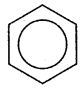Question 17.
Define resonance (mesomeric) effect.
The permanent polarity is produced by the interation of lone pair & π electrons in conjugate a system of an organic molecule.

Question 18.
Indicate the type of electron pair involved in M effect.
π-electron pair or lone pair electrons.

Question 19.
What is +R effect ?
Shift of electron pair away from substituent in congjuagte system.

Question 20.
Define inductive effect.
The permanent polarity in an saturated organic molecule due to small displacement of sigma bond pair of electrons towards more electronegative atom.Question 21.
Which electron displacement effect involves displacement of s-electrons?
Sigma bond pair of electrons.

Question 22.
What is +1 effect ?
Small displacement of sigma bond pair of electrons away from substituent.

Question 23.
Give an example of a group which exerts +1 effect.
Alkyl group (methyl group)

Question 24.
Out of (CH3)2 CH – and CH3 – group, which is a better electron releasing group?
(CH3)2CH-

Question 25.
What is -1 effect ?
Small displacement of sigma bond pair of electrons towards substituent.

Question 26.
Give an example of electron withdrawing group.
Nitro group (-NO2)

Question 27.
Identify the stronger electron withdrawing substituent from each of the following pairs.

1. -NO2 and -Cl
2. -C ≡ N and -Br
3. -Cl and -OCH3
4. -C6H5 and -F

1. -N02
2. -C ≡ N
3. -Cl
4. -F

Question 28.
What is R-effect?
Shift of electron pair towards the substituent in a conjugate system.

Question 29.
What is Inductive effect?
The polarisation of one σ bond caused by polarization of adjacent a bond due to difference in electro-negativity.

Question 30.
Sketch the resonance structure of Benzene.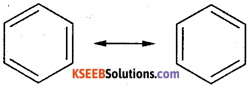Question 31.
What is the suitable adsorbent in the process of column chromography?
Al3O3 (alumina)

Question 32.
Which process is used to purify impure sample or camphor, contaminated with sand?
Sublimation

Question 33.
Which gas is liberated in Kjeldahl’s method?
Ammonia gas (NH3)

Question 34.
What is Lassaigne’s extract ?
When organic compound is fused with sodium metal and then extracted by water, it is called Lassaigne’s extract.

Question 35.
Which gas is liberated in Dumas method?
N2

Question 36.
Define Crystallization.
The process of getting crystals when hot saturated solution of a compound is cooled is called crystallization.

Question 37.
What is basic principle of chromotography ?

• Paper,
• Fhinlayer,
• Column

Question 38.
What is steam distillation ?
The distillation which is carried out with the help of steam is called steam distillation.

Question 39.
Suggest the method to purify:
(a) Camphor containing salt impurity
(b) Kerosene Oil containing water
(c) Mixture of Benzene and Toulene
(d) Sugar and Naphtalene
(a) sublimation
(b) by solvent extraction
(c) fractional Distillation
(d) treat with water and filter

Question 40.
Why is fusion of an organic compound with sodium required ?
It is done so as to convert organic compound into inorganic compound.Question 41.
Name two classes of compounds in which Kjeldahl’s method cannot be used for estimation of nitrogen.
Nitro compounds, Azo compounds and compounds containing nitrogen in ring, e.g., pyridine.

Question 42.
Which class of compounds are tested with the help of Beilstein test.
Halogen containing organic compounds but some other compounds like urea is respond to this test.

Question 43.
What are formulae of

• Sodium nitroprusside
• Ferric ferrocyanide ?

• Na2[Fe(CN)5NO]
• Fe2[Fe(CN)6]3

Question 44.
Which elements are estimated by Liebig’s Method?
Carbon and Hydrogen

Question 45.
What is the relationship between molecular mass and equivalent mass of an acid?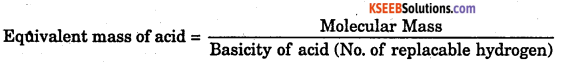Question 46.
What is relationship between molecular formula and empirical formula of a substance ?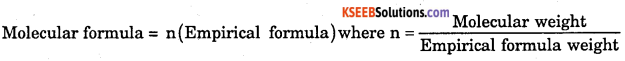Question 47.
Which effect involves the displacement of electron pair under the infulence of an attacking reagent ?
Electromeric effect.

Question 48.
What is an electromeric effect ?
The shift of π electron pair of a multiple bond to one of the bonded atoms during the attack of electrophile or nucleophile.

Question 49.
Which type of E effect Operates during the attack of proton on ethane molecule ?
+E effect.

### 1st PUC Chemistry Organic Chemistry: Some Basic Principles and Techniques Two Marks Questions and Answers

Question 1.
What is Electrophile ? Give example.
The electron deficient molecules or positively charged ions which are capable of accepting on electron from substrate molecule are called electrophiles.
Positive electrophiles : H+, Cl+, Br+, NO2, N+ = O, R+ (carbocations) etc.
Neutral electrophiles : SO3, BF3, AICl3, Cl

Question 2.
What are Nucleophiles ?
The molecules or negatively charged ions which are capable of donating an electron pair to electron deficient centre of the substrate are called nucleophiles.
Negative nucleophiles: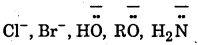Question 3.
What is the difference between organic and inorganic compounds ?

 Property Organic Compounds Inorganic Compounds Chemical nature Compounds of carbon Compounds of elements other than carbon Bonding Covalent Ionic Melting . and boiling points Low, generally Volatile High, generally non-­volatile Solubility Soluble in organic solvents, insoluble in water. Soluble in water, insoluble in organic solvents. Electrical conduction Non – conductors ; solutions and liquids – non – electrolytes Conductors ; solutions and liquids – conductors

Question 4.
What is the difference between Electrophilic Reagents and Nucleophilic Reagents.

 Electrophilic Reagents Nucleophilic Reagents 1. These are electron loving species. 1. These are nucleus loving species 2. These are electron deficient species. 2. These are electron rich species. 3. They attack the site of high electron density in the substrate. 3. They attack the site of low electron density in the substarte. 4. They may be +vely charged ions (cations) or neutral molecules. 4. They may be negatively charged ions (anions) or neutral molecules. 5. They possess an atom with incomplete octet. 5. They possess an atom withlone pair of electrons. 6. They are Lewis acids. 6. They are Lewis bases. 7. They accept an electron pair from the substrate to form a covalent bond. 7. They done an electron pair to the substrate to form a covalent bond.

Question 5.
What is Homolytic fission (Homolysis) ? Give reason.
Definition: The fission of a covalent bond, in which each of the two species produced, retains one electron of shared electron pair, is called hemolytic fission or homolysis,
Eg: $$\mathrm{Cl}-\mathrm{Cl} \stackrel{\text { Homolysis }}{\longrightarrow} 2 \mathrm{Cl}^{-}$$ (Chloroine free radicals)Question 6.
What is Heterolytic Fission (Heterolysis)?
The fission of a covalent bond, in which ionic species are produced due to unequal disrtribution of bonded electron pair, is called heterolytic fission or heterolysis.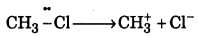Question 7.
What is Homolytic Fission and Heterolytic Fission?

 Homolytic Reagent Heterolytic Fission 1. In this case, the covalent bond breaks symmetrically. 1. In this case, the covalent bond breaks unsymmetrically. 2. Each species, obtained, retains one electron from shared electron pair. 2. The more electronegative otom retains the shred electron pair. 3. Electrically neutral free radicals are formed which carry an old certain. 3. Electrically charged ions (cations and anions) are formed. 4. It takes place in the presence of sunlight, U.V. light or by pyrolysis. 4. It takes place in the presence of polar solvent.

Question 8.
What are Free Radical ? Give example.
A free radical can be defined as an atom or group of atoms having an odd or impaired electron. Putting a dot (•) against the symbol of atom or group of atoms.
Example :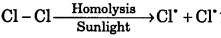(Chlorine free radicals)

Question 9.
What are the characteristics of Free Radicals.

• Free radicals are generally electrically neutral.
• They carry on odd (unpaired) electron.
• They are highly unstable.
• They are short-lived.
• They are highly reactive species due to the presence of odd electrons.
• They have a tendency to pair up with the odd electrons to form a covalent bond.
• They are generally formed either in presence of U.V. or visible light or in the presence of peroxides.

Question 10.
What is Alicylic compounds? Give Example.
These are saturated hydrocarbons joined by covalent bond to form ring structure.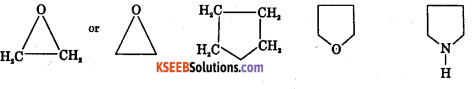Question 11.
What are Aromatic Compounds ? Give example.
These are the compounds containing atleast one benzene ring.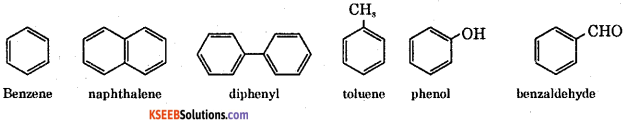Question 12.
What are Heterocyclic compound? Give example.
These are the compounds containing ring structure in which one or more carbon atoms are replaced by hetero atoms such as N, S, O etc.,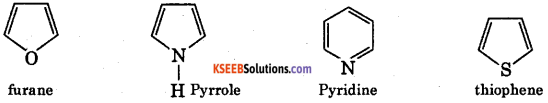Question 13.
What is functional group? Give example.
A functional group is defined as “an atom or group of atoms present in a molecule which determines most of the chemical properties of the particular class of organic compounds”.
Example : CH3 – OH methyl alcohol.

Question 14.
What are carbanions ? Give example.
A reaction intermediate formed by heterolysic fission of a covalent bond which contains one negatively charged carbon with eight electrons in its valence shell is called carbanion.
The heterolytic cleavage of a covalent bond as indicated in the following reactions gives carbanions.
Example : $$\mathrm{CH}_{3}-\mathrm{MgI} \longrightarrow \mathrm{CH}_{3}^{-}+\mathrm{M}^{+} \mathrm{gI}$$

Question 15.
What are carbocations ? Give Example.
A reaction intermediate formed by heterolytic fission of a covalent bond which contains one positively charged carbon with three bond pair electrons (sextext of electrons) is called carbocation.
The heterolytic fission of bromomethane yields methyl carbocation and bromide ion as shown below.
Example :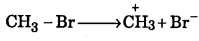Question 16.
What is the difference between Carbocation & Carbanion.

 Carbocation Carbanion 1. The central carbon atom is +vely charged. 1. The central carbon atom is -vely charged. 2. It is an electron deficient species. 2. It is an electron rich species. 3. The central carbon atom possesses six electrons in its outermost shell. 3. The central carbon atom possesses eight electrons in its outermost shell. 4. The central carbon atom is in sp2 state of hybridisation. 4. The central carbon atom is in sp3 state of hybridisation and carries a lone pair of electrons. 5. Its shape is triangular planner. 5. Its shape is pyramidal. 6. It can accept an electron pair from a nucleophile to form a covalent bond. 6. It can donate an electron pair to an electrophile to form a covalent bond.

Question 17.
What is positive inductive effect (+1 effect) ? Give example.
In this effect the substituent (Y) releases electron pair away from itself. In other words a bond pair of electrons are displaced away from the substituent.
Eg : All alkyl groups.Question 18.
What is negative inductive effect (-1 effect) ? Give example.
In this effect, the sigma bond pair of electrons are displaced towards electron withdrawing substituent (X).
The order of electron withdrawing ability (intensity of -1 effect) of a few substituent is given below.

Question 19.
What is electromeric effect ? Give example.
It is the complete transfer of shared pair of electrons of a multiple bond to one of the atom in the presence of attacking reagent.
Eg.: H+, -CN, etc.,

Question 20.
What is +E effect ? Give example.
When the transfer of electrons takes place towards the attacking reagent, the effect is called +E effect.
For example, the addition of an acid to alkenes.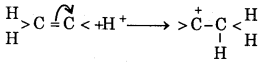Question 21.
What is -E Effect ? Give example.
When the transfer of electron takes place way from the attacking reagent, the effect is called -E effect.
For example, the addition of cyanide ion (CN-) to carbonyl group (>C = 0)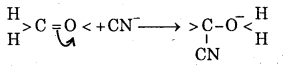Question 22.
What is mesomeric effect ? Give example.
The polarity developed in a molecule, as a result of interaction between two π-bond or a π-bond and a lone pair of electrons is referred to as mesomeric effect.
Groups with +M or +R effect.
—Cl, —Br, —I, -NH2, -NHR, -NR2, -OH, -OR, -SH, -OCH3 etc.,
Groups with -M or -R effect.
-NO2, -C ≡ N, -C-, -CHO, -COOH, -COOR etc.,

Question 23.
What is the difference between Inductive effect & Mesomeric effect?

 Inductive Effect Mesomeric Effect 1. It operates in saturated compounds. 1. It operates in unsaturated compounds especially having conjugated systems. 2. It involves electrons of a – bonds. 2. It involves electrons of π – bonds or lone pair of electrons. 3. The electron pair is slightly displace from its position and hence partial charges are developed. 3. The electron pair is completely transferred and hence unit positive and negative charges are developed. 4. It is transmitted over a short distance it becomes negligible after second carbon atom in the chain. 4. It is transmitted through the entire chain provided conjugation is present.

Question 24.
What is the difference between Inductive Effect and Electromeric Effect.

 Inductive Effect Electromeric Effect 1. It is permanent in nature. 1. It is temporary in nature. 2. It is due to electronegative atom present in the molecule itself. 2. It is due to approach of the attacking reagent. 3. It is the mobility of electrons along C-C single bond. 3. It is the mobility of electrons in a multiple bond (double or triple bond).

Question 25.
What is resonance energy ? Mention the resonance energy of Benzene.
The phonomenon in which two or more structures can be written for a compound but none of them represents it correctly is called resonance. The actual structure of the compound is said to be a resonance hybrid. 36 kcal mol-1 is the resonance energy of benzene.

Question 26.
Explain hyperconjucgation effect.
The electron release of alkyl group bonded to unsaturated system in which delocalization of electrons takes place through overlap between C – H sigma (σ) orbital and pi (π) bond orbited or vacant p-orbital is known as hyperconjugation.

Question 27.
What is substitution reaction ? Give example.
The reaction in which an atom of group atoms attached to carbon atom in a substrate molecule, is replaced another atom is called substitution reaction.
Ex: $$\mathrm{CH}_{4}+\mathrm{Cl}_{2} \stackrel{\mathrm{UV}}{\longrightarrow} \mathrm{CH}_{2} \mathrm{Cl}+\mathrm{HCl}$$

Question 28.
What is addition reaction ? Give example.
The reaction in which the attacking reagent adds up to the substrate molecule without elimination of any molecule are called addition reaction.
Ex :
$$\mathrm{CH} \equiv \mathrm{CH}+\mathrm{H}_{2} \frac{\mathrm{Ni}}{14: \mathrm{C}} \cdot \mathrm{CH}_{2}=\mathrm{CH}_{2}$$

Question 29.
What is the test for sulphur ?
To a small amount of sodium extract, freshly prepared solution of sodium nitro prusside is added, a deep violet colour indicates the presence of sulphur.
Ex: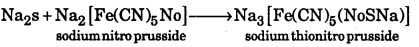### 1st PUC Chemistry Organic Chemistry: Some Basic Principles and Techniques Three/Four Marks Questions and Answers

Question 1.
How is the detection of carbon and hydrogen by copper oxide test ?
Organic compounds undergo oxidation in the presence of a suitable oxidizing agent. In this process, carbon is oxidized to CO2 and hydrogen is oxidized to water.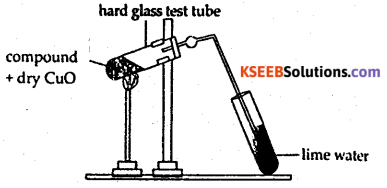Procedure : The compound is initially lime water mixed with dry cupric oxide. The mixture is strongly heated in a hard glass test, tube fitted with a cork and a delivery tube. The liberated gases are passed into lime water. Carbon present in compound is oxidised by cupic oxide to carbon
dioxide, which turns lime water milky. The hydrogen present in the compound is converted into water which turns anhydrous copper sulphate to blue hydrated salt.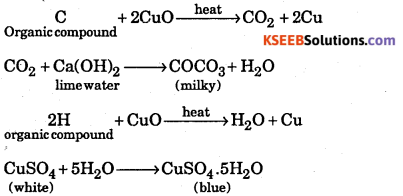Question 2.
How is the prepartion on of Lassaigne’s is filtrate ?
Procedure : A piece of dry sodium is introduced into a fusion tube and heated till it melts. A drop of few crystals of the organic compound is added to the fusion tube. The mixture is fused gently on a Bunsen flame initially. The tube is then heated until red hot and plunged into in a mortar containing distilled water. The contents are ground throughly and filtered. The filtrate is known as sodium fusin extract, stock solution or Lassaigne’s extract. The filtrate is divided into three parts, which are used for the detection of nitrogen, sulphur and halogen in organic compound.

Question 3.
How is detection of nitrogen by Lassaigne’s filtrate ?
A few crystal of ferrous sulphate are added to the first part of the filtrate. The mixture is boiled and cooled. It is acidified with hydrochloric acid and a few drops of ferric chloride solution are added. Sodium cyanide in the filtrte reacts with ferrous sulphate to give sodium ferrocyanide. It further reacts with ferric chloride to give a blue coloured solution of ferric ferrocyanide.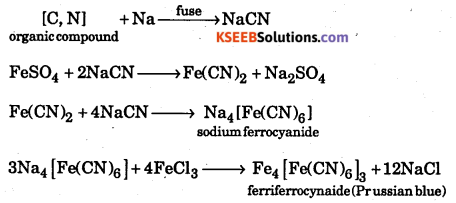Question 4.
How is the detection of sulphur by Lassaigne’s is filtrate ?
Lead acetate test: second part of the filtrate is treated with excess of acetic acid and lead acetate solution. A black precipitate of lead sulphide is formed.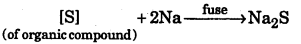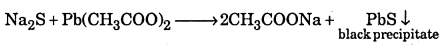Question 5.
Explain the test for detection of halogens by Lassaigne’s is filtrate
Silver nitrate test : A portion of the stock solution is boiled with dil. HN03, cooled and silver nitrate is added. A white precipitate soluble in ammonium hydroxide shows the presence of chlorine. A pale yellow precipitate slightly in ammonium hydroxide shows the presence of bromine. A yellow precipitate insoluble in ammonium hydroxide ” shows the presence of iodine.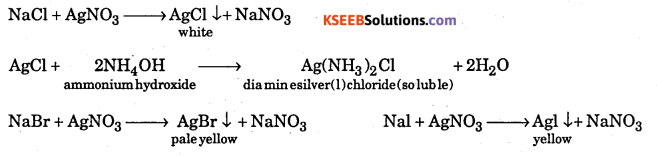Question 6.
Explain the test for detection of phs phosphorus in an organic compound.
Organic compound containing phosphorous is fused with sodium peroxide. The
phosphorus of the compound is oxidised to phosphate. The fused mass is extracted with water and filtered. The filtrate containing sodium phosphate is boiled with nitric acid and then treated with ammonium molybdate. A yellow solution of precipitate indicates the presence of phosphorus.

Question 7.
Explain the estimation of phosphorus in organic compound by carius method.
It is also estimated by carius method the known mass of organic compound containing phosphorus is heated with fuming HNO3 when phosphorus is oxidised to phosphoric acid (H3PO4). To this, magnesia mixture (MgSO4 + NH4OH + NH3CI) is added when phosphoric acid precipitates as magnesium ammonium phosphate (MgNH4PO4). This precipitate is filtered, washed, dried and ignited when it is converted to magnesium pyrophosphate (Mg2P2O3).
$$2 \mathrm{MgNH}_{4} \mathrm{PO}_{4} \stackrel{\Delta}{\longrightarrow} \mathrm{Mg}_{2} \mathrm{P}_{2} \mathrm{O}_{7}+2 \mathrm{NH}_{3}+\mathrm{H}_{2} \mathrm{O}$$
The weight of Mg2P2CO7 is determied from which the percentage of phosphorus is the compound can be calculated.
Observations and Calculations:

1. Mass of organic compound taken = w1, g.
2. Mass of Mg2P2O7 obtained = W2g.

We have $$\underset{222 \mathrm{g}}{\mathrm{Mg}_{2} \mathrm{P}_{2} \mathrm{O}_{7}} \equiv \underset{62 \mathrm{g}}{2 \mathrm{P}} \text { Now, } 222 \mathrm{g} \mathrm{Mg}_{2} \mathrm{P}_{2} \mathrm{O}_{7}$$contains 62g of phosphorus.
W2g of Mg2p2O7 Will contain $$\frac{62 \times w_{2}}{222} g$$ of phosphorus
This amount of phosphorus was present in wig of the compound
$$\therefore \% \mathrm{P}=\frac{62 \times \mathrm{w}_{2}}{222} \times \frac{100}{\mathrm{w}_{1}}$$Question 8.
0.189g of an organic substance containing chlorine gave carius method
0. 287g of silver chloride. Calculate the percentage of chlorine in the substance.

1. Mass of organic compound taken (W1) = 0.189 g
2. Mass of AgCli ppt (W2) = 0.287 g

Now , %$$\mathrm{Cl} 2=\frac{35.5 \times \mathrm{w}_{2}}{143.5} \times \frac{100}{\mathrm{w}_{1}}=\frac{35.5 \times 0.287 \times 100}{143.5 \times 0.189}=37.57$$

Question 9.
0.2632g of silver bromide is obtained from 0.2562g of an organic compound. Find the percentage of bromine in the compound.

1. Mass of organic compound (W1) = 0.2562g
2. Mass of AgBr obtained (W2) = 0.2632g.
$$\text { Now } \% \mathrm{Br}_{2}=\frac{80 \times \mathrm{w}_{2}}{188} \times \frac{100}{\mathrm{w}_{1}}=\frac{80 \times 0.2632 \times 100}{188 \times 0.2562}=43.71$$

Question 10.
In Leibig’s method. 0.24 g of organic compound on combustion with dry oxygen produced of 0.62 g of CO2 and 0.11 g of II2O. Determine the percentage composition of the compound.
Mass of organic compound = m = 0.24 g .
Mass of carbon dioxide formed = 0.62 g
Mass of water formed = 0.11 g
Percentage of carbon = $$\frac{12}{4} \times \frac{0.62}{0.24} \times 100=70.45$$
Percentage of hydrogen = $$\frac{2}{4} \times \frac{0.11}{0.24} \times 100=5.09$$
Percentage of oxygen = [100 – (70.5 + 5.0)] = 24.46

Question 11.
In Carius method of estimation of halogen, 0.20 g of organic compound gave 0.15 g of silver bromide. Calculate the percentage of bromine in the compound.
Mass of organic compound (m1) = 0.20 g
Mass of silver bromide formed (m2) = 0.15 g
188 (108 + 80) g of AgBr contains 80 g of bromine
∴ 0.20 g of AgBr contains = $$\frac{80 \times 0.15}{188} \mathrm{g}=0.0638 \mathrm{g} \text { of } \mathrm{Br}$$
Percentage of bromine = $$\frac{80 \times 15 \times 100}{188 \times 20}=31.92$$
The percentage of bromine in the given compound = 31.92

### 1st PUC Chemistry Organic Chemistry: Some Basic Principles and Techniques Five Marks Questions and Answers

Question 1.
Describe with a neat diagram the estimation of carbon and hydrogen by Leibig’s method.
Principle : A known mass of an organic compound is strongly heated with dry cupric oxide (CuO), when carbon and hydrogen are quantitatively oxidized to CO2 and H2O respectively. The masses of CO2 and H2O thus formed are determined. From this, the percentages of carbon and hydrogen can be calculated.
Procedure : Pure and dry oxygen is passed through the entire assembly of the apparatus (Fig) till the CO2 and moisture is completely removed.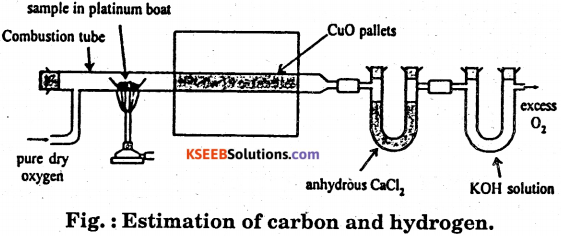A boat containing weighed organic substances is introduced inside from one end of the combustion tube by opening it for a while. The tube is now strongly heated till the whole of the organic compound is burnt up. The flow of oxygen is continued to drive CO2 and water vapours completely to the U-tubes. The apparatus is cooled and the U-tubes are weighed separately.
Observed and Calculations.

1. Mass of organic compound taken = w.g.
2. Mass of water produced = x g (Increase in mass of CaCk tube)
3. Mass of carbon dioxide produced = y g. (Increase in mass of KOH tube)

To determine % of carbon
Molar mass of CO2 = 44g mol-1
Now, 44g of CO2 = contains 12 g of C.
∴ y g of CO2 will contain of $$\frac { 12y }{ 44 }$$ g of C.
This amount of carbon was present in w. g. of the substance
$$\therefore \% \mathrm{C}=\frac{12 \mathrm{y}}{44} \times \frac{100}{\mathrm{w}}$$
To determine % of Hydrogen
Molar mass of water = 18 g mol-1
Now 18g of H2O contains 2 g of H2
∴ x g of H2O will contain $$\frac { 2x }{ 18 }$$ g of H2
This amount of hydrogen was present in weight of substance.
$$\therefore \% \mathrm{H}_{2}=\frac{2 \mathrm{x}}{18} \times \frac{100}{\mathrm{w}}$$

Question 2.
How is the estimation of Nitrogen in ogranic compound by Dumas method.
Principle : The organic compound containing nitrogen when heated with excess of copper oxide in the atmosphere of carbon dioxide, yields nitrogen in addition to carbon dioxide and water.

Traces of nitrogen oxides formed during combustion of organic compound are reduced to nitrogen by passing the gaseous mixture over a heated copper gauze. The percentage of nitrogen present in a given organic compound is calculated from the volume of nitrogen collected over potassium hydroxide solution from a known mass of organic compound.

Procedure : The apparatus used for the estimation of nitrogen by this method is shown in the figure.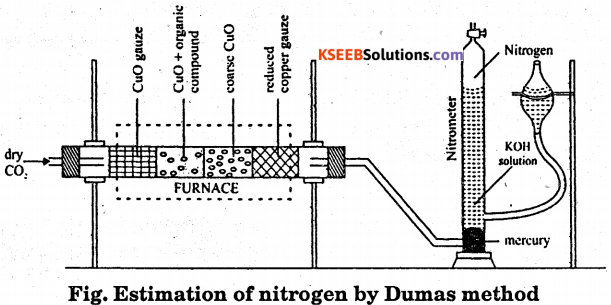A known mass of organic compound is mixed with copper oxide and placed in the combustion tube. The carbon dioxide gas is passed through the combustion tube to displace air present in the tube. The combustion tube is now heated in the furnace, the nitrogen evolved collects in the nitrometer. The volume of the nitrogen collected is recorded after adjusting the levels of potassium hydroxide solution in the two limbs are equal. Room temperature and atmosphere pressure are recorded.
Calculation:
Mass of organic compound = mg
Volume of nitrogen in nitrometer = V cm3
Room temperature = t° C = (273 + t) K
Atmosphere pressure = P1 mm
Aqueous tension at room temperature = P’ mm
Pressure of dry nitrogen gas formed = P = (P – P’) mm
Volume of nitrogen at STP (V0) = $$\frac{\mathrm{PV} \times 273}{760 \times(273+\mathrm{t})} \mathrm{cm}^{3}$$
22,400 cm3 of nitrogen of STP = 28 g of nitrogen
Mass of V0 cm3 of nitrogen = $$\frac{28 \times V}{22,400} g$$
Percentage of nitrogen = $$\frac{28 \times V_{0} \times 100}{22,400 \times m}$$Question 3.
Describe an experiment to determine the percentage of nitrogen in an organic compound by Kjeldahl’s method.
Principle : When a nitrogenous organic compound is heated with cone. H2SO4 using (CUSO4K2SO4) as a catalyst, the nitrogen from the compound is quantitatively converted to ammonium sulphate.

This ammonium sulphate is decomposed by heating with excess of alkali and the ammonia evolved is absorbed in known excess of a standard solution of H2SO4. Part of acid is neutralized by ammonia. The excess of acid left behind after neutralization with ammonia is estimated by back titration with standard alkali. From this, the amount of acid actually consumed by ammonia can be obtained which can be used to determine the percentage of nitrogen in the compound.

Procedure : A known exact mass of organic compound (about 0.5g) ix mixed with lOg K2SO4, Ig CuSO4 and 25 ml of cone. H2SO4. The mixture is heated strongly in a Kjeldahl’s flask. Till the contents become clear. This step is known as digestion.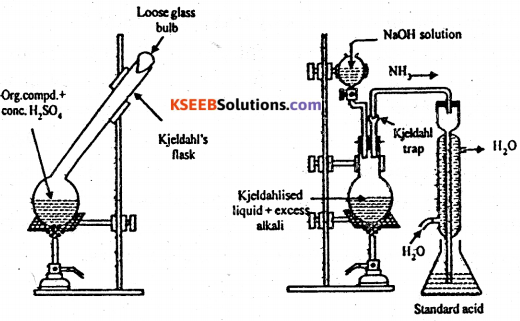The Kjeldahl’s flask is now cooled and the liquid is heated in a round-bottomed flask with excess of caustic soda solution The ammonia evolved is absorbed in a known volume of a standard acid.
The amount of unreacted acid is determined by titrating it against a standard alkali.
(NH4 )2S04 + 2NaOH → 2NH3 ↑+ Na2S04 + 2H20
Observation :

1. Mass of organic compound taken = Weight
2. Normality of standard acid = N
3. Volume of standard acid taken = V1 ml
4. Volume of alkali (Normality = N) required for back titration = V2 ml

Calculation: Volume of acid used up by ammonia = Volume of ammonia produced
= (V2 – V1) = V ml of normality N.
Now,
1000 ml of 1 normal NH3 = 17g NH3 = 14g N2
∴ Vml of N- normal ammonia will contain $$\frac{14 \times \mathrm{N} \times \mathrm{V}}{1000} \mathrm{gN}_{2}$$
This amount of nitrogen was present in w g of the compound
$$\therefore \% \mathrm{N}_{2}=\frac{14 \times \mathrm{N} \times \mathrm{V}}{1000} \times \frac{100}{\mathrm{w}} \text { or } \% \mathrm{N}_{2}=\frac{1.4 \mathrm{NV}}{\mathrm{w}}$$
Where, N and V are the normality and volume respectively of the acid used up by ammonia.

Question 4.
How is the estimation of halogens by Carius method ?
When an organic compound containing halogen (Cl, Br or 1) is heated in a sealed tube with fuming nitric acid and excess of silver chloride, silver halide is formed from the mass of silver halide obtained, the percentage of the halogen can be calculated.
Procedure : In a hard glass tube (Carius tube), 5ml of fuming HNO3 and 2 to 2.5 g AgNO3 are taken. A small narrow weighing tube, containing a small amount (nearly 0.15-0.2g) of accurately weighed organic compound, is introduced in the Carius tube in – such a way that nitric acid does not enter the weighing tube. The Carius tube is now sealed and heated in a furnace at 300°C for about six hours.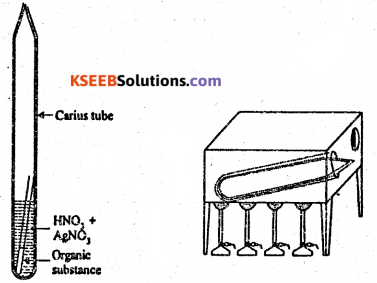The tube is then cooled and its narrow end is cut off and the contents are completely transferred to a beaker by washing with water. The precipitate of silver halide formed is filtered through a weighed sintered glass crucible. It is washed, dried and weighed. Observation and calculation :

1. Mass of organic compound taken = W1g
2. Mass of silver halide obtained = W2 g

(a) For chlorine :
$$\underset{143.5 \mathrm{g}}{\mathrm{AgCl}} \equiv \underset{35.5 \mathrm{g}}{\mathrm{Cl}}$$
143.5g of AgCl contains 35.5 g of chlorine
w2g of Agcl Will contain $$\frac{35.5 \times w_{2}}{143.5} g$$ of chlorine
This amount of chlorine was present in wig of the compound.
$$\therefore \quad \% \mathrm{Cl}_{2}=\frac{35.5 \times \mathrm{w}_{2}}{143.5} \times \frac{100}{\mathrm{w}_{1}}$$

(b) For bromine :
$$\underset{188 \mathrm{g}}{\mathrm{AgBr}} \equiv \underset{80 \mathrm{g}}{\mathrm{Br}}$$
188g of AgBr contains 80g of bromine
W2 g of AgBr will contain $$\frac{980 \times w_{2}}{188} g$$ of bromine.
$$\therefore \% \mathrm{Br}_{2}=\frac{80 \times \mathrm{w}_{2}}{188} \times \frac{100}{\mathrm{w}_{1}}$$

(c) For Iodine :
$$\underset{235 \mathrm{g}}{\mathrm{Agl}} \equiv \underset{127 \mathrm{g}}{\mathrm{I}}$$
235g of Agl contains 127g of iodine
W2 g of Agl will contain $$\frac{127 \times w_{2}}{235} g$$ of iodine
$$\therefore \quad \% \mathrm{I}_{2}=\frac{127 \times \mathrm{w}_{2}}{235} \times \frac{100}{\mathrm{w}_{1}}$$